Question

# capacitors are connected as shown to a potential difference of V=14.0 volts. CisC,-Cr=C,-C,-20 μ1: The charge...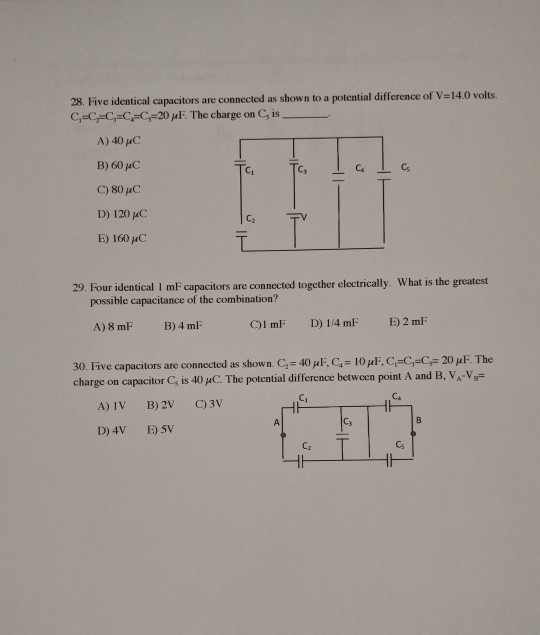capacitors are connected as shown to a potential difference of V=14.0 volts. CisC,-Cr=C,-C,-20 μ1: The charge on C5 is A) 40 pC B) 60 HC C) 80 uC D) 120 HC E) 160 μC C1 C3 C2 29·Four identical 1 mF capacitors are connected together electrically what is the greatest possible capacitance of the combination? A) 8 mF B) 4 mF 30 Five capacitors are connected as shown. C2 40 μ F, C4# 10 μ1, Ci=C,sCF 20 μ F The charge on capacitor Cs is 40 μ(. The potential difference between point A and B, VA-VB- Cz Cs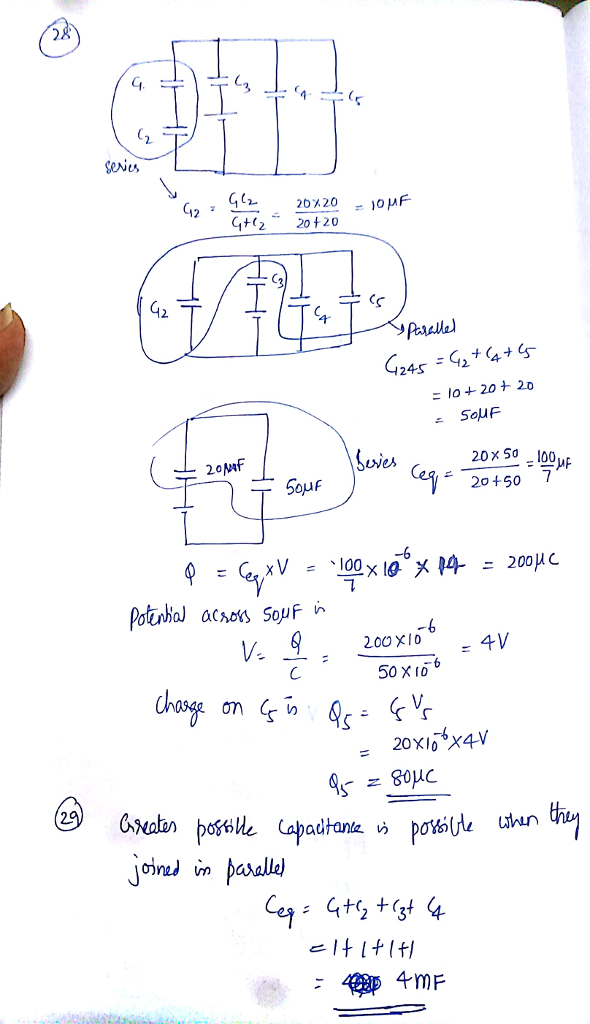#### Earn Coins

Coins can be redeemed for fabulous gifts.

Similar Homework Help Questions
• ### A circuit is constructed with five capacitors and a battery as shown. The values for the...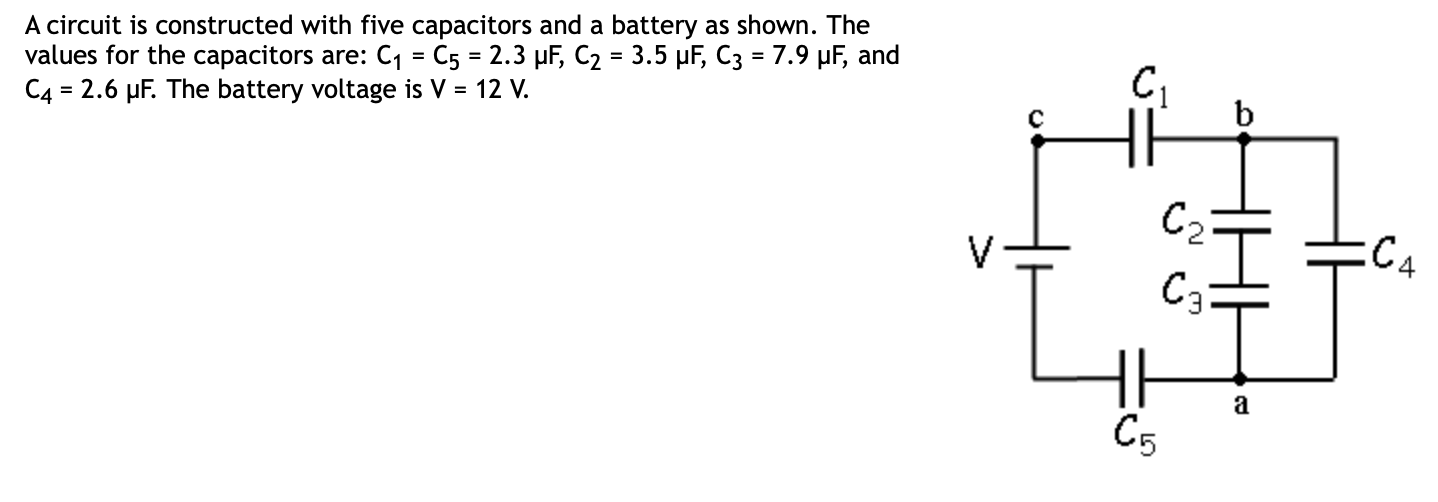A circuit is constructed with five capacitors and a battery as shown. The values for the capacitors are: C1 = C5 = 2.3 uF, C2 = 3.5 uF, C3 = 7.9 pF, and C4 = 2.6 uF. The battery voltage is V = 12 V. EC4 4) What is Q2, the charge on Cz? C Submit 5) What is Q1, the charge on capacitor Cy? uc Submit 6) What is V4, the voltage across capacitor C4? Submit

• ### In the diagram below, C1=30 uF, C7=15 MF, Cz=10 uF, C=20 MF, and C=10 pF. Find...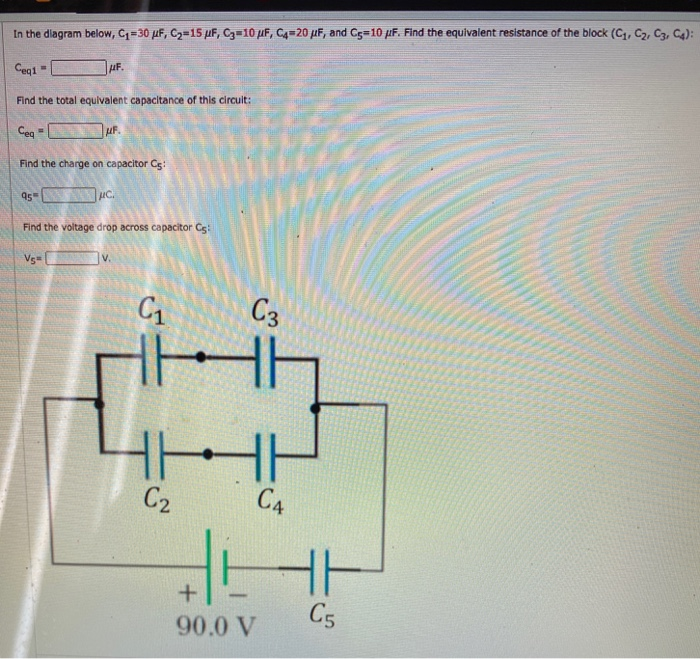In the diagram below, C1=30 uF, C7=15 MF, Cz=10 uF, C=20 MF, and C=10 pF. Find the equivalent resistance of the block (C1, C2, C3, C4): Cegt MF. Find the total equivalent capacitance of this circuit: Ceg UF Find the charge on capacitor Cs: 95-ENAC. Find the voltage drop across capacitor Cs Vse С. C3 HE HA C2 C4 HE + 90.0 V C5

• ### A circuit is constructed with five capacitors and a battery as shown. The values for the...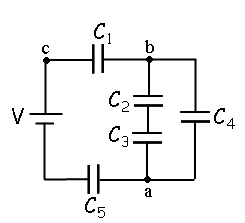A circuit is constructed with five capacitors and a battery as shown. The values for the capacitors are: C1 = C5 = 4.2 μF, C2 = 1 μF, C3 = 5.8 μF, and C4 = 4.4 μF. The battery voltage is V = 12 V. 1) What is Cab, the equivalent capacitance between points a and b?μF 2) What is Cac, the equivalent capacitance between points a and c? 3) What is Q5, the charge on capacitor C5?μC 4) What...

• ### Fig. 1 shows a circuit of four capacitors connected to a battery with a potential difference...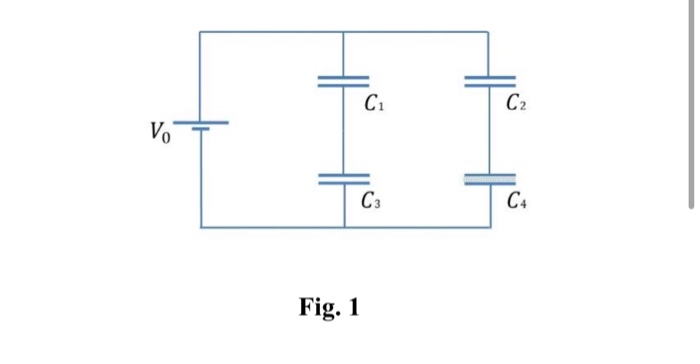Fig. 1 shows a circuit of four capacitors connected to a battery with a potential difference ?_0. Capacitor 4 is filled with a dielectric with 0 dielectric constant ? = 4. The capacitance value of the capacitors is equal ?1 =?2 = ?3 = ?. The capacitance of capacitor 4 is also equal to ? / 2 when in the middle of their plates there is no dielectric. a) Calculate the free charge stored in the capacitor 4. Its answer...

• ### Find the total capacitance C total of the combination of capacitors shown in the figure, where...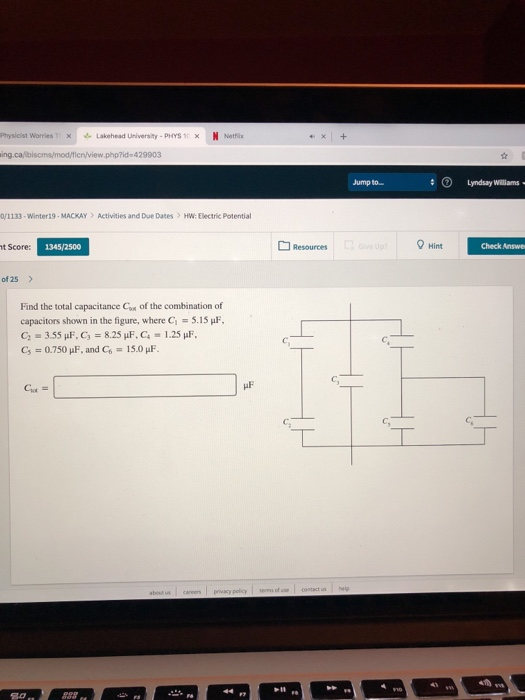Find the total capacitance C total of the combination of capacitors shown in the figure, where C1= 5.15 uF C2=3.55uF, C3=8.25uF , C4=1.25uF , C5 =0.750 uF and C6 = 15.0uF Physicist worries T ×、 f, Lakehead University . PHYS 1: x N Nletfix ing.calbiscms/mod/fien/view.php?id-429903 Jump to. Lyndsay Williams 0/1133-Winter19- MACKAY Activities and Due Dates HW: Electric Potential Resources Hint t Score: 1345/2500 Check Answe of 25 > Find the total capacitance Cx of the combination of capacitors shown in...

• ### In the circuit shown on the right, with all capacitors fully charged:  the potential difference...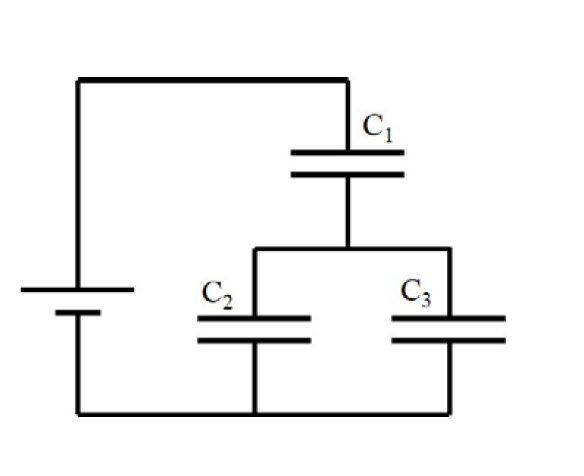In the circuit shown on the right, with all capacitors fully charged:  the potential difference across C1 is equal to 5.0 V  the charge stored on C1 is equal to 2.5 μC  the charge stored on C2 is equal to 1.2 μC  the capacitance of C3 is equal to 0.65 μF Calculate the total equivalent capacitance of the circuit. 3 2

• ### 20 of 22 > Four capacitors are arranged in the circuit shown in the figure. The...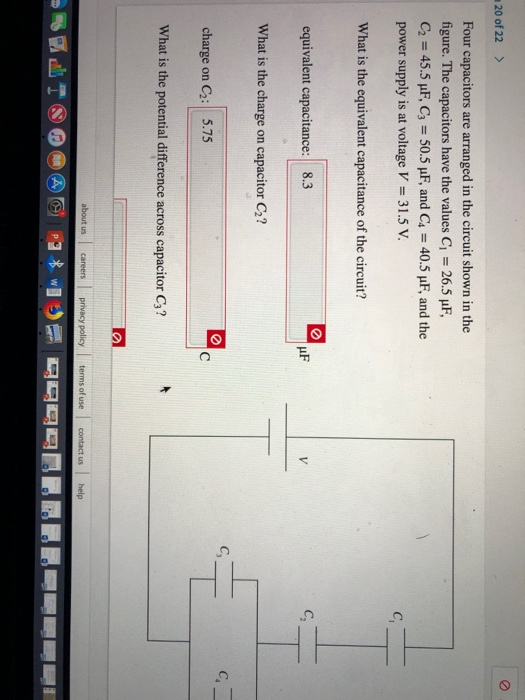20 of 22 > Four capacitors are arranged in the circuit shown in the figure. The capacitors have the values C 26.5 uF, C2 45.5 uF, C3 50.5 pF, and C4 40.5 uF, and the power supply is at voltage V 31.5 V C What is the equivalent capacitance of the circuit? C equivalent capacitance: 8.3 V What is the charge capacitor C2? on C. charge C2: 5.75 C on What is the potential difference across capacitor C3? about us...

• ### Question 10 3 pts A capacitor has a small capacitance of C. The capacitor is charged...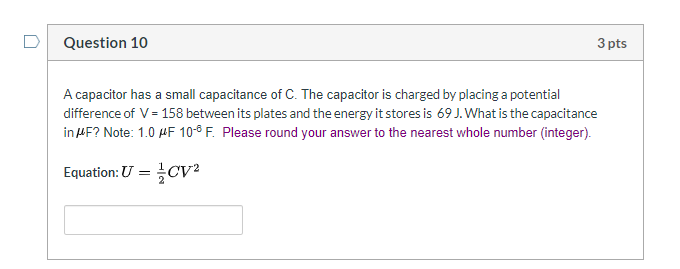Question 10 3 pts A capacitor has a small capacitance of C. The capacitor is charged by placing a potential difference of V = 158 between its plates and the energy it stores is 69 J. What is the capacitance in MF? Note: 1.0 MF 10- F. Please round your answer to the nearest whole number (integer). Equation: U = CV Question 11 3 pts The figure below shows a circuit that contains four capacitors. Find (a) the equivalent capacitance,...

• ### The circuit in the figure below shows four capacitors connected to a battery. The switch s...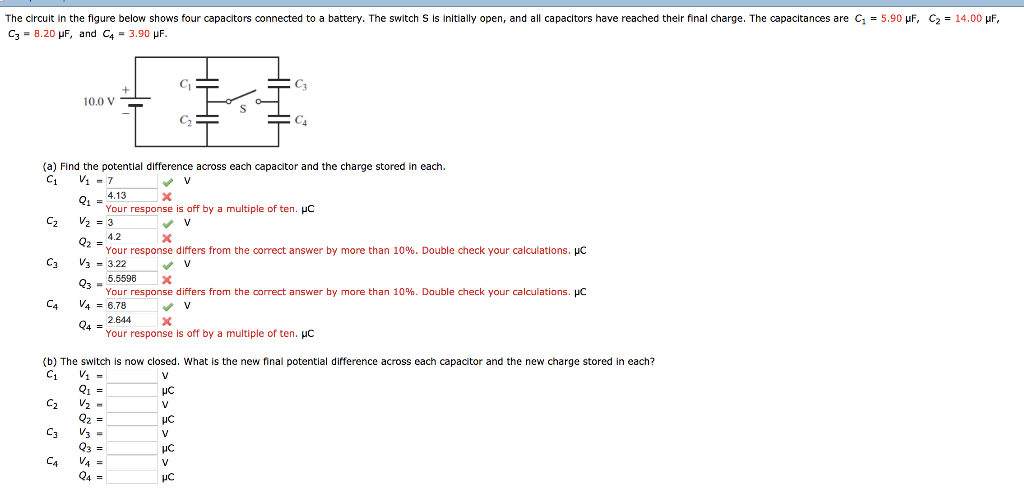The circuit in the figure below shows four capacitors connected to a battery. The switch s is initially open, and all capacitors have reached their final charge. The capacitances are C1 = 5.90 μF, C3 = 8.20 μF, and C4 = 3.90 μF C2 = 14.00 μF 10.0 V (a) Find the potential difference across each capacitor and the charge stored in each. 4.13 Your response is off by a multiple of ten. HC 4.2 Your response differs from the...

• ### Problem 2: A 1KO resistor is connected with a 20 mF capacitor and a battery of...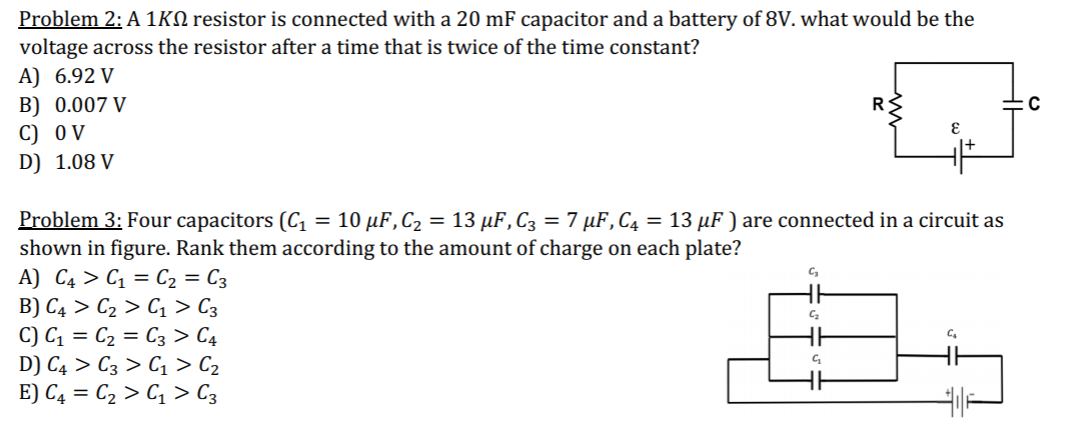Problem 2: A 1KO resistor is connected with a 20 mF capacitor and a battery of 8V. what would be the voltage across the resistor after a time that is twice of the time constant? A) 6.92 V C B) 0.007 V C) OV D) 1.08 V Problem 3: Four capacitors (C1 = 10 uF,C2 = 13 uF, C3 = 7 uF,C4 = 13 uF) are connected in a circuit as shown in figure. Rank them according to the amount...